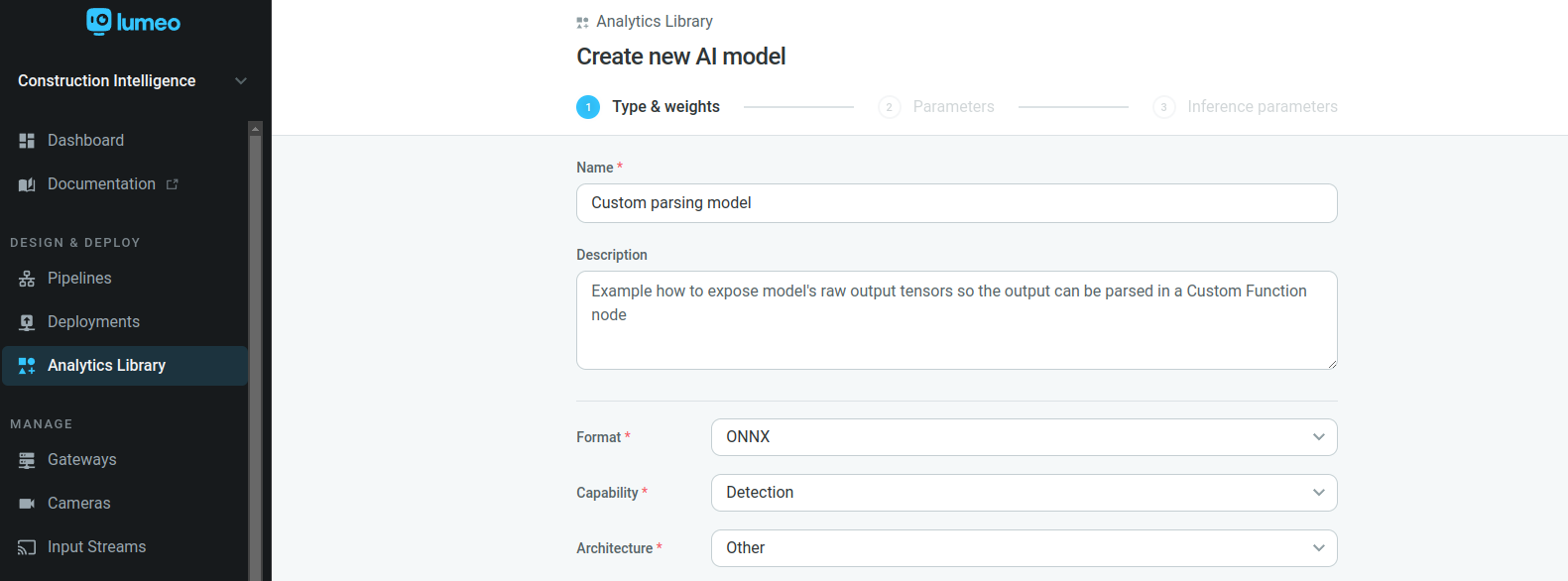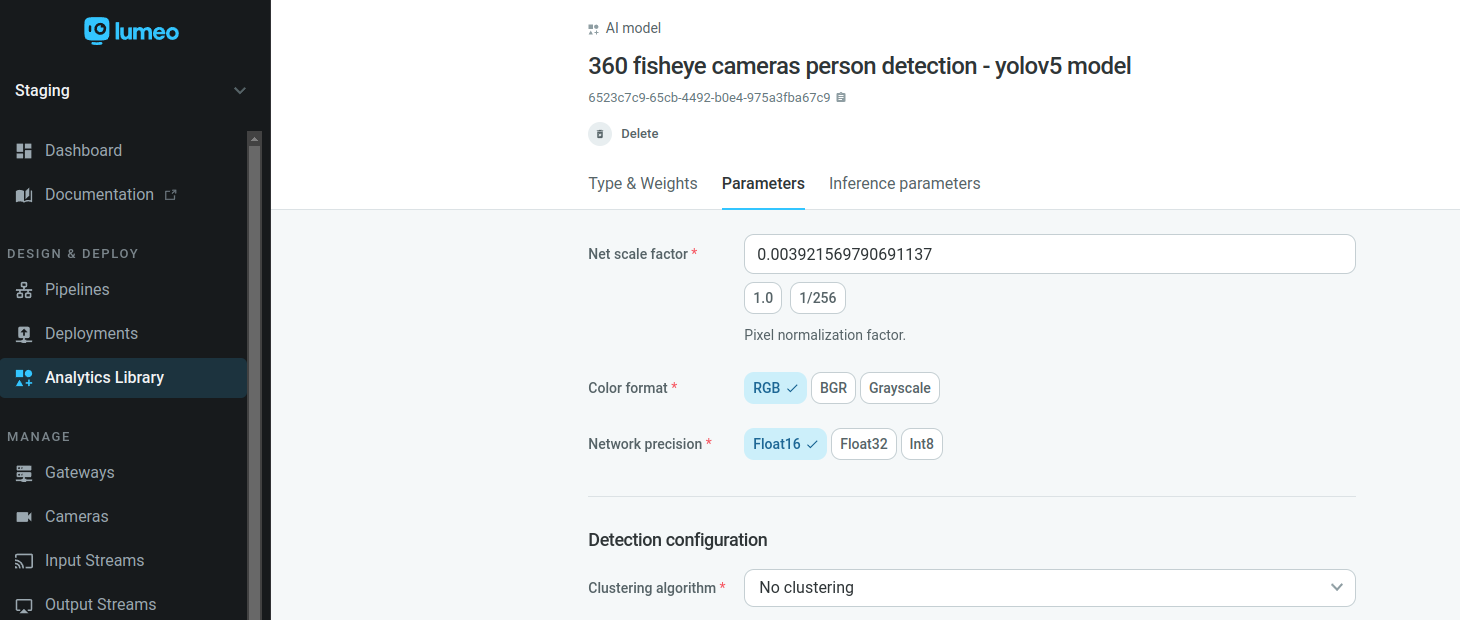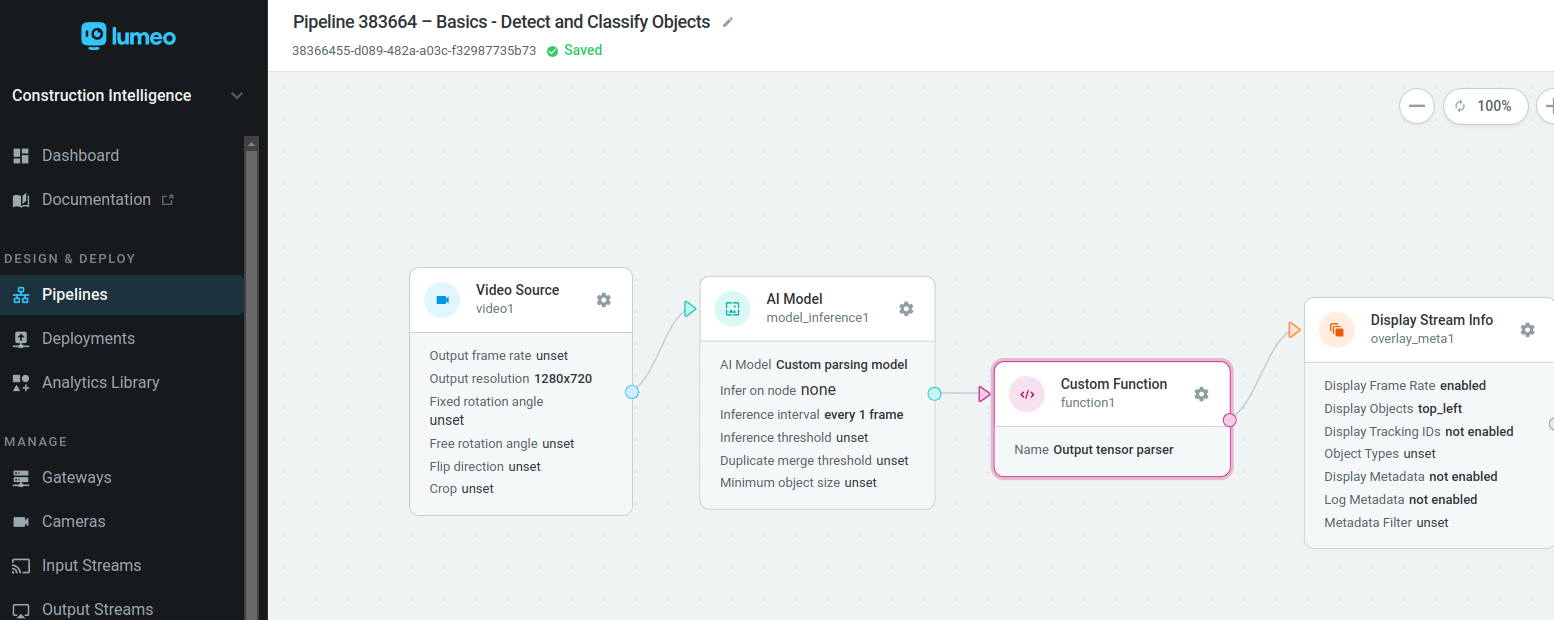# Custom Model Parser

We are continuously improving the inference support for different model's formats and architectures.

There might be some cases were we don't support parsing the inference output of some custom or less known architectures, but you can access the output tensor raw results and parse them in a Function Node.

Below we share the required steps in the model & pipeline configuration, and a snippet of the python code that can be used to access the raw tensors, parse them and store the results in the frame metadata.

# 1) Configure the inference model to disable internal parsingSelect "Architecture -> Other" in model "Type & weights" tabSelect "No clustering" on the "Clustering algorithm" of "Parameters" tab

# 2) Add a Custom Function node to process inference raw output tensorsConnect a "Custom Function" after the "AI Model" node

# 3) Write the python code in the "Custom Function" to parse the inference output and store the results in frame metadata

``````from lumeopipeline import VideoFrame
import cv2
import numpy as np

# Global variables that persist across frames go here.
# One time initialization code can also live here.

def process_frame(frame: VideoFrame, deployment_id=None, node_id=None, **kwargs) -> bool:

with frame.data() as mat:

# Get the inference model raw output tensor(s)
tensors = frame.tensors()
print("Tensors length = {}".format(len(tensors)))

# Variables where we will store the output tensor as numpy array
heatmaps = pafs = None

for tensor in tensors:
# If there are multiple upstream inference nodes, it might be required filter the tensor by
# the corresponding inference node 'source_node_id'
print("tensor.source_node_id = {}".format(tensor.source_node_id))

for layer in tensor.layers:
print("  Layer name = {}".format(layer.name))
print("  dimensions = {}".format(layer.dimensions))
print("  data = {}".format(layer.data))

# Copy the raw tensor data, filtering by output tensor 'layer.name'
if layer.name == "output_conf:0":
heatmaps = np.asarray(layer.data.copy()).reshape(layer.dimensions)
elif layer.name == "output_paf:0":
pafs = np.asarray(layer.data.copy()).reshape(layer.dimensions)

# Get the height and width of the frame
height, width, _ = mat.shape

if heatmaps is not None and pafs is not None:

###### This step might not be required for your model
# Just an example how to resize the output tensors to half of the size of the input image
heatmaps = np.transpose(heatmaps, (1, 2, 0))
heatmaps = cv2.resize(heatmaps, (int(width/2), int(height/2)), interpolation=cv2.INTER_CUBIC)

pafs = np.transpose(pafs, (1, 2, 0))
pafs = cv2.resize(pafs, (int(width/2), int(height/2)), interpolation=cv2.INTER_CUBIC)
######

current_poses = []
# Insert here the code to parse the output tensors and extract meaningful information (detected objects, classifier results, etc)
#
# Tracking logic and object clustering (for example NMS / Non-maximum Suppression) can also be applied here.

# Save the metadata on Lumeo frame

return True

try:
# Access frame metadata
meta = frame.meta()

# Get the "objects" field
objects = meta.get_field("objects")

# Iterate over the detected objects on this frame, and create a new object (or update existing ones)
for pose in current_poses:
pose_obj = {
"label": "person",
"class_id": 0,
"probability": pose.confidence,
"rect": {
"left": pose.bbox,
"top": pose.bbox,
"width": pose.bbox,
"height": pose.bbox,
},
}
objects.append(pose_obj)

# Save results on Lumeo frame metadata, so it can be access later in downstream nodes
meta.set_field("objects", objects)
meta.save()

except Exception as error:
print(error)
pass
``````